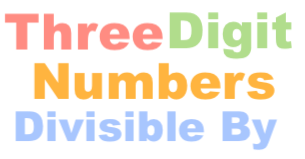Three Digit Numbers Divisible By CalculatorThe Three Digit Numbers Divisible By Calculator can calculate all the three digit numbers that can be divided by a number.

In other words, you have a number and you want to know all the 3-digit numbers that when divided by your number will result in a whole number.

Here are some examples of what this calculator can answer:

How many three digit numbers are divisible by 13?

3-digit numbers divisible by 11

How many three digit numbers are divisible by 7?

3-digit numbers divisible by 8

Three digit numbers divisible by 5

How many three digit numbers are divisible by 9?

How many three digit numbers are divisible by 3?

3-digit numbers divisible by 12

How many three digit numbers are divisible by 6?

How many 3-digit numbers are divisible by 5?